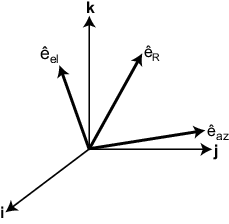# azelaxes

Spherical basis vectors in 3-by-3 matrix form

## Syntax

``A = azelaxes(az,el)``

## Description

example

````A = azelaxes(az,el)` returns a 3-by-3 matrix containing the components of the basis$\left({\stackrel{^}{e}}_{R},{\stackrel{^}{e}}_{az},{\stackrel{^}{e}}_{el}\right)$ at each point on the unit sphere specified by azimuth, `az`, and elevation, `el`. The columns of `A` contain the components of basis vectors in the order of radial, azimuthal and elevation directions.```

## Examples

collapse all

At the point located at 45° azimuth, 45° elevation, compute the 3-by-3 matrix containing the components of the spherical basis.

`A = azelaxes(45,45)`
```A = 3×3 0.5000 -0.7071 -0.5000 0.5000 0.7071 -0.5000 0.7071 0 0.7071 ```

The first column of `A` contains the radial basis vector `[0.5000; 0.5000; 0.7071]`. The second and third columns are the azimuth and elevation basis vectors, respectively.

## Input Arguments

collapse all

Azimuth angle specified as a scalar in the closed range [–180,180]. Angle units are in degrees. To define the azimuth angle of a point on a sphere, construct a vector from the origin to the point. The azimuth angle is the angle in the xy-plane from the positive x-axis to the vector's orthogonal projection into the xy-plane. As examples, zero azimuth angle and zero elevation angle specify a point on the x-axis while an azimuth angle of 90° and an elevation angle of zero specify a point on the y-axis.

Example: `45`

Data Types: `double`

Elevation angle specified as a scalar in the closed range [–90,90]. Angle units are in degrees. To define the elevation of a point on the sphere, construct a vector from the origin to the point. The elevation angle is the angle from its orthogonal projection into the xy-plane to the vector itself. As examples, zero elevation angle defines the equator of the sphere and ±90° elevation define the north and south poles, respectively.

Example: `30`

Data Types: `double`

## Output Arguments

collapse all

Spherical basis vectors returned as a 3-by-3 matrix. The columns contain the unit vectors in the radial, azimuthal, and elevation directions, respectively. Symbolically we can write the matrix as

`$\left({\stackrel{^}{e}}_{R},{\stackrel{^}{e}}_{az},{\stackrel{^}{e}}_{el}\right)$`

where each component represents a column vector.

collapse all

### Spherical basis

Spherical basis vectors are a local set of basis vectors which point along the radial and angular directions at any point in space.

The spherical basis vectors $\left({\stackrel{^}{e}}_{R},{\stackrel{^}{e}}_{az},{\stackrel{^}{e}}_{el}\right)$ at the point (az,el) can be expressed in terms of the Cartesian unit vectors by

`$\begin{array}{ll}{\stackrel{^}{e}}_{R}\hfill & =\mathrm{cos}\left(el\right)\mathrm{cos}\left(az\right)\stackrel{^}{i}+\mathrm{cos}\left(el\right)\mathrm{sin}\left(az\right)\stackrel{^}{j}+\mathrm{sin}\left(el\right)\stackrel{^}{k}\hfill \\ {\stackrel{^}{e}}_{az}\hfill & =-\mathrm{sin}\left(az\right)\stackrel{^}{i}+\mathrm{cos}\left(az\right)\stackrel{^}{j}\hfill \\ {\stackrel{^}{e}}_{el}\hfill & =-\mathrm{sin}\left(el\right)\mathrm{cos}\left(az\right)\stackrel{^}{i}-\mathrm{sin}\left(el\right)\mathrm{sin}\left(az\right)\stackrel{^}{j}+\mathrm{cos}\left(el\right)\stackrel{^}{k}\hfill \end{array}.$`

This set of basis vectors can be derived from the local Cartesian basis by two consecutive rotations: first by rotating the Cartesian vectors around the y-axis by the negative elevation angle, -el, followed by a rotation around the z-axis by the azimuth angle, az. Symbolically, we can write

`$\begin{array}{ll}{\stackrel{^}{e}}_{R}\hfill & ={R}_{z}\left(az\right){R}_{y}\left(-el\right)\left[\begin{array}{c}1\\ 0\\ 0\end{array}\right]\hfill \\ {\stackrel{^}{e}}_{az}\hfill & ={R}_{z}\left(az\right){R}_{y}\left(-el\right)\left[\begin{array}{c}0\\ 1\\ 0\end{array}\right]\hfill \\ {\stackrel{^}{e}}_{el}\hfill & ={R}_{z}\left(az\right){R}_{y}\left(-el\right)\left[\begin{array}{c}0\\ 0\\ 1\end{array}\right]\hfill \end{array}$`

The following figure shows the relationship between the spherical basis and the local Cartesian unit vectors.## Algorithms

MATLAB® computes the matrix `A` from the equations

```A = [cosd(el)*cosd(az), -sind(az), -sind(el)*cosd(az); ... cosd(el)*sind(az), cosd(az), -sind(el)*sind(az); ... sind(el), 0, cosd(el)];```

## Version History

Introduced in R2013a